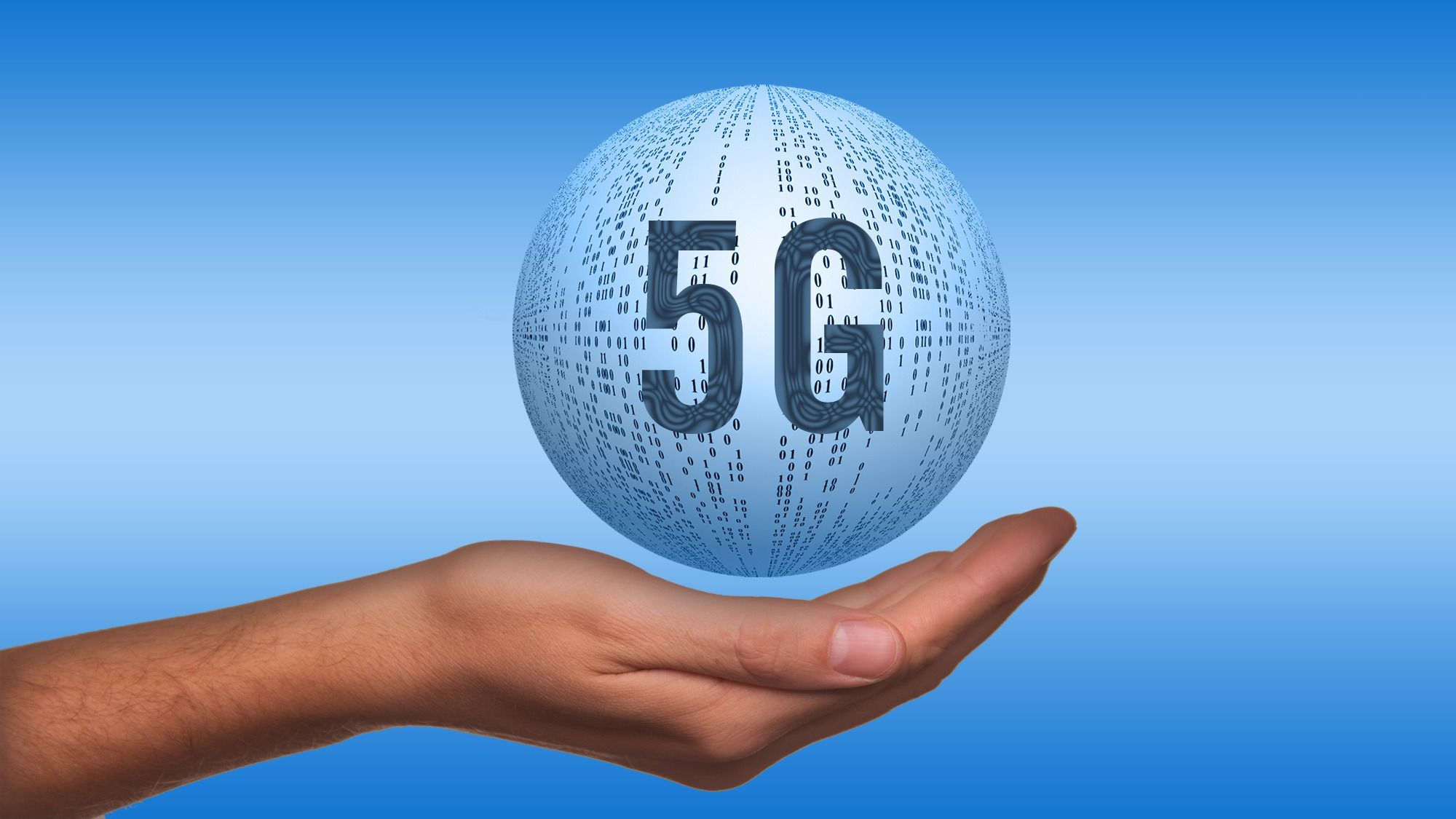BlogFirst and foremost, consumers must realize what’s involved with 30 GHz. A Gigahertz (GHz) is a frequency equal to one billion hertz or cycles per second. Now multiply that 30 times; how many cycles per second do you get? Isn’t that 30 billion cycles per second? Now, imagine those GHz frequencies traveling over in-wall copper electric house wires that are built to take only 60 Hz!

Such GHz frequencies just may be considered as sinusoidal harmonics or “dirty electricity.”

To put the above into proper perspective about microwave technology and non-ionizing radiation waves such frequencies emit, we need to understand the frequency of electricity. One Hertz (Hz) equals one cycle per second. One kilohertz (KHz) is equivalent to one thousand cycles per second. One megahertz (MHz) equals one million cycles per second. One gigahertz (GHz) is equal to one billion hertz or cycles per second—frequencies not found naturally in Nature, except from man-made/generated electromagnetics.